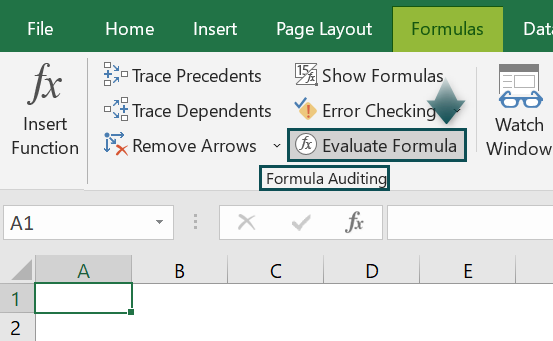# Evaluate Formula in ExcelArticle byExcelMojo Team## What is Evaluate Formula in Excel?

Evaluate Formula in Excel tool is used to understand the formula’s working. This feature works on each argument in a formula to recognize and secure any errors that occur.

The Evaluate Formula assists us in evaluating complex formulas and examining each part of a nested function to calculate the final result.

For example, we will calculate the data’s total using the MAX IF Excel Formula using the EVALUATE FORMULA Option.

The steps to evaluate the MAX & IF formula using the EVALUATE FORMULA are as follows:

1. Select empty cells for the output. We have selected cell B7 in this case.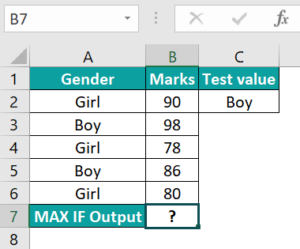2. Next, we will enter the MAX & IF formula in cell B7.

3. Enter the condition as A2:A6=C2, B2:B6.

4. The complete formula is =MAX(IF(A2:A6=C2,B2:B6)) in cell B7.

5. Press Enter key. The result is obtained in cell B7 as 98.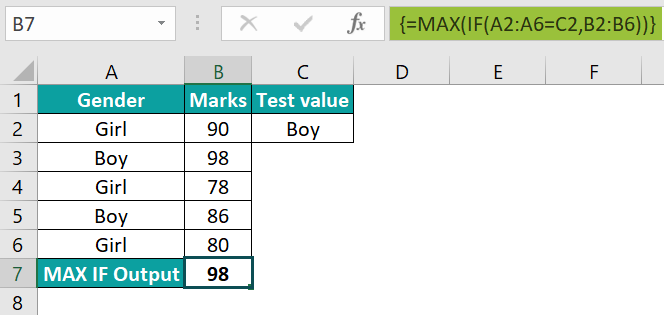6. Select the Evaluate Formula option from the Formula Auditing in the Formulas tab.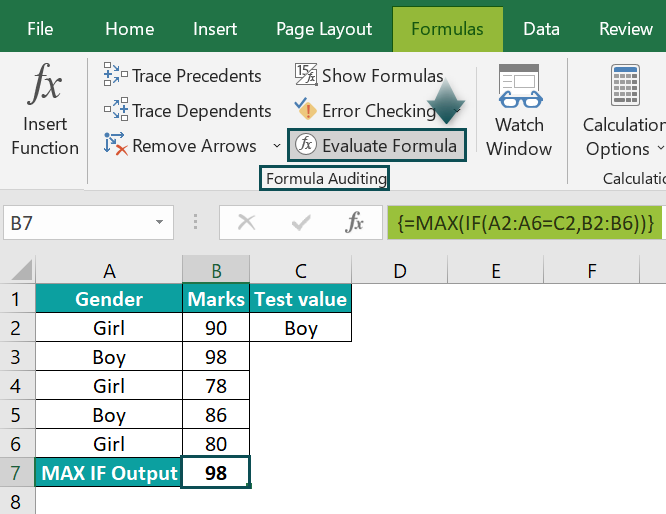7. Select the formula cell in the worksheet.

8. The window called Evaluate Formula pops up.

9. The formula is shown in the Evaluation box.

10. Select the Evaluate button to start the evaluation of the formula.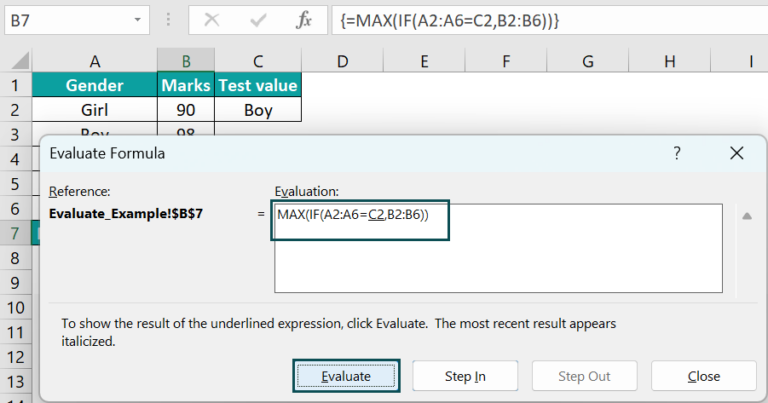11. The evaluated result is shown in the Evaluation box.

12. Select the Close button to close the evaluation. We can also select the Restart button to evaluate the formula.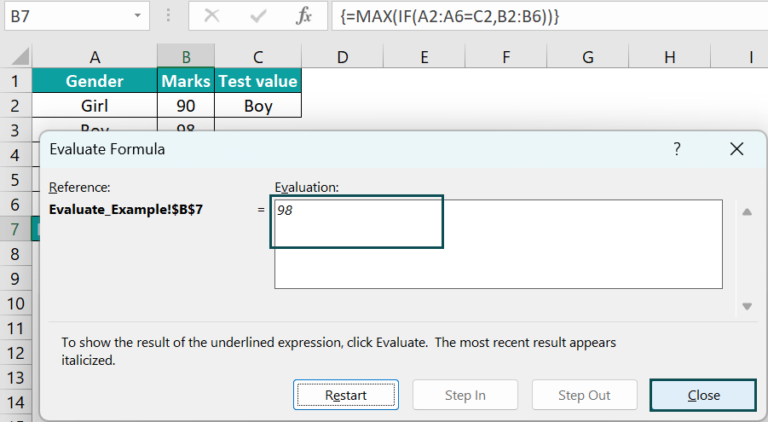###### Key Takeaways
• Evaluate Formula in Excel tool is used to understand how a formula is functioning.
• The Evaluate Formula assists us in evaluating complex formulas and examining each part of a nested function to calculate the final result.
• Evaluate Formulas in Excel evaluates each argument in the formula and finds errors.
• The PEMDAS is used to Evaluate Formulas in Excel.
• The shortcut of the Evaluate Formula in Excel is CTRL + ~ (tilde).
• We can also press F9 key to evaluate formula in excel.

### Explanation and Uses

There are many calculations and logical tests to evaluate the nested formula calculation. The Evaluate Formula shows the different parts of a nested formula in the dialog box and evaluates in the order the formula is calculated.

To evaluate the formula uses the F2 key for toggling between Edit and Enter modes in Excel. To change the formula, select the cell containing the formula and press F2 to enter the Edit mode. The cursor points at the end of the closing parenthesis in the cell. The left and right arrows are used to navigate within the formula. To shift to select the formula parts, the arrow keys are used. Click the Delete or Backspace keys to delete the cell references or elements of the formula. Press Enter to complete the formula for editing. To exit the Edit mode, press the Esc key without changing the other modes. Here, we will show a quick, simple, and effective way to fix formulas and functions in Excel.

The Evaluate Formula saves hours; it is the tool that will help to troubleshoot the formula. The formula that contains a cell references a cell on the worksheet; Excel adjusts those cell references automatically when a copy or moves the cell that contains the formula, no matter where a copy or move it within the worksheet or workbook.

### How to Evaluate Formula in Excel?

#### #1 – Evaluate Excel Formula Using F9 Key

We will use IF Excel Formula using F9 KeyIF Function tests whether the condition is fulfilled and returns the result as “true” and “false” for the logical text or value.

The syntax of the IF Function is; =IF(logical_test,value_if_true,value_if_false).

For example, the below image shows values, and we will calculate the logical condition of the given data using the IF Excel Formula using the F9 Key.

In the table,

• Column A: contains Values.
• Column B: contains Output.

The steps to evaluate the formula using the IF function are as follows:

• Step 1:  Select empty cells for the output. We have selected cell B2 in this case.
• Step 2:  Next, we will enter the IF formula in cell B2.
• Step 3: Enter the condition as A2<100.
• Step 4: The complete formula is =IF(A2<100“True”,“False”) in cell B2.
• Step 5: After entering each value in the preceding step, press the Enter key. The result is obtained as True in cell B2.
• Step 6: Press Enter and drag the cursor to cell B3, as shown in the following image.

If we do not understand the formula, we can evaluate it by pressing the F9 key.

• Step 7: The F9 key is used to evaluate quickly and fix the formula to understand and proficiently work in Excel. The F9 key is used to determine only the part of the formula by replacing the actual formula with the result of the formula.

Note: The above example shows the logical test used in the formula, i.e., A2<100. We detect the status by evaluating the logical test. If the value is <100, then the result is “True,” or else the result is “False.” When the condition tests return “True,” that means the logical test is “TRUE,” and if the logical test is “FALSE,” it will return “False.”

#### #2 – Example

For example, the below image shows values, and we will calculate the logical condition of the given data using the IF Excel Formula using the F9 Key.

In the table,

• Column A: contains Name.
• Column B: contains Output.

The steps to evaluate the formula using the IF function are as follows:

• Step 1:  Select empty cells for the output. We have selected cell B2 in this case.
• Step 2:  Next, we will enter the IF formula in cell B2.
• Step 3: Enter the condition as A2= “John”.
• Step 4: The complete formula is =IF(A2= “John”,“True”) in cell B2.
• Step 5: Press Enter key. The result in cell B2 is returned as TRUE in cell B2.
• Step 6: Press Enter and drag the cursor to cell B3, as shown in the following image.

If we do not understand the formula, we can evaluate it by pressing the F9 key.

• Step 7: The F9 key is used to evaluate quickly and fix the formula to understand and proficiently work in Excel. The F9 key is used to determine only the part of the formula by replacing the actual formula with the result of the formula.

Note: The above example shows the logical test used in the formula, i.e., =“John.” We detect the status by evaluating the logical test. If the name is =“John,” then the result is “True,” or else the result is “False.” When the condition tests return “True,” that means the logical test is “TRUE,” and if the logical test is “FALSE,” it will return “False.”

#### #3 – Evaluate Formula by Using EVALUATE FORMULA Option

We will use SUM Excel Formula using EVALUATE FORMULA OptionSUM Function enables users to add individual numeric values, cell references, ranges, or all three together. The Auto Sum function in Excel, i.e., “” automatically adds all the numeric values listed in a particular row or column.

The syntax of the SUM Function is; =SUM(number1,[number2],…).

For example, we will calculate the data’s total using the SUM Excel Formula using the EVALUATE FORMULA Option.

In the table,

• Column A: contains Numbers.
• Column B: contains Output.

The steps to evaluate the SUM formula using the EVALUATE FORMULA are as follows:

• Step 1:  Select empty cells for the output. We have selected cell B2 in this case.
• Step 2:  Next, we will enter the SUM formula in cell B2.
• Step 3: Enter the condition as A2:A10.
• Step 4: The complete formula is =SUM(A2:A10) in cell B2.
• Step 5: Press Enter key. The result is returned in cell B2 as shown in the image below.
• Step 6: Select the Evaluate Formula option from the Formula Auditing in the Formula tab.
• Step 7: Select the formula cell in the worksheet.
• Step 8: The window called the Evaluate Formula pops up.
• Step 9: The formula is shown in the Evaluation box.
• Step 10: Select the Evaluate button to start the evaluation of the formula
• Step 11: The evaluated result is shown in the Evaluation box.
• Step 12: Select the Close button to close the evaluation. We can also select the Restart button to evaluate the formula.

### Important Things to Note

• In this article, we discuss How to Use Evaluate Formula Using F9 Key
• Press the Esc key when evaluating the formula.
• The “#REF!” error occurs when it contains multiple cells.
• The excel shortcut keys ALT + M + V allows us to evaluate the formula cell by cell.

1. What is the Shortcut of the Evaluate Formula in Excel?

The Shortcut of the Evaluate Formula in Excel is as follows;
CTRL + ~ (tilde)
F9 key is also used to Evaluate the Formula.

2. What rule does the Evaluate Formula in Excel use?

The Evaluate Formula uses operators for specific calculations that we apply to the elements of a formula. The Evaluate Formula in Excel follows general mathematical calculation rules: Parentheses, Exponents, Multiplication and Division, and Addition and Subtraction, as the PEMDAS.

3. Where is the Evaluate Formula in Excel?

One can activate the Evaluate Formula in Excel using the following steps:
1) Choose the formula cell which contains the result.
2) Go to the Formulas tab and click it.
3) Select Evaluate Formula from the Formula Auditing group.
4) A window called Evaluate Formula appears.
5) The formula is shown in the Evaluation box.
6) Select the Evaluate button to start the evaluation of the formula.
7) Select the Close button to close the evaluation.